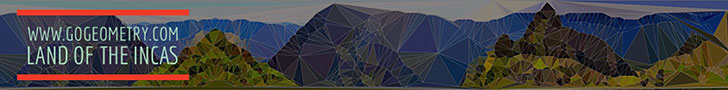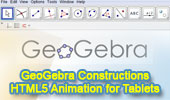# Dynamic Geometry Software: GeoGebraGeoGebra - IndexDynamic Geometry 1481. Five Tangential or Circumscribed Quadrilaterals, Pitot Theorem, Congruence, Step-by-step Illustration. GeoGebra, iPad. Dynamic Geometry 1480. Japanese Theorem for Cyclic Polygon, Sangaku, Triangulation, Non-intersecting Diagonals, Sum of Inradii, Invariant, Step-by-step Illustration. GeoGebra, iPad. Dynamic Geometry 1479. Triangle, Circumcircle, Angle Bisector, Perpendicular Bisector, Chord, Concyclic Points, Parallel Lines,, Step-by-step Illustration. GeoGebra, iPad. Dynamic Geometry 1478. Cyclocevian, Reuschle-Terquem Theorem, Concurrent Cevians, Triangle, Circumcircle, Secant line, Step-by-step Illustration. GeoGebra, iPad. Dynamic Geometry 1477. Miquel's Pentagram Theorem, Pentagon, Triangle, Circumcircles, Concyclic Points, Step-by-step Illustration. GeoGebra, iPad. Dynamic Geometry 1476. Droz-Farny Line Theorem, Triangle, Orthocenter, Perpendicular, Collinear Midpoints, Step-by-step Illustration. GeoGebra, iPad. Dynamic Geometry 1475. Clifford Intersecting Circles Theorem, Step-by-step Illustration, GeoGebra, iPad. Dynamic Geometry 1474. Butterfly Theorem, Circle, Chords, Midpoints, Step-by-step Illustration. Dynamic Geometry 1473. Kosnita's Theorem, Triangle, Four Circumcenters, Concurrent Line, Step-by-step Illustration. Dynamic Geometry 1472. Cyclic Quadrilateral, Perpendicular Diagonals, Rectangle, Step-by-step Illustration. Dynamic Geometry 1471. Equilateral Triangle, Inside/Outside Point, Incenters, Tangency Points, Concurrent Lines, Step-by-step Illustration. Dynamic Geometry 1470. Tangential Quadrilateral, Incircles, Tangent, Parallel, Rhombus, Step-by-step Illustration. Dynamic Geometry 1469. Triangle, Circumradius, Inradius, Midpoints, Arcs, Sum of Distances, Step-by-step Illustration. Dynamic Geometry 1468. Steiner's Theorem, Triangle, Circumradius, Inradius, Sum of Exradii, Step-by-step Illustration. Geometry Problem 1466. Tangential Quadrilateral, Newton Line, Incenter, Midpoint, Diagonal, Collinear Points. Step-by-step Illustration using GeoGebra. Geometry Problem 1465. Tangential Quadrilateral, Incenter, Inscribed Circle, Equal Sum of Areas. Step-by-step Illustration using GeoGebra. Geometry Problem 1464. Quadrilateral, Interior Point, Midpoint of Sides, Equal Sum of Areas. Step-by-step Illustration using GeoGebra. Geometry Problem 1463. Parallelogram, Interior Point, Opposite Triangles with Equal Sum of Areas. Step-by-step Illustration using GeoGebra. Geometry Problem 1462. Newton-Line, Newton-Gauss Line, Complete Quadrilateral, Midpoints of Sides and Diagonals, Collinear Points. Dynamic Geometry 1460. Newton-Gauss Line, Complete Quadrilateral, Midpoints of Diagonals, Collinear Points, Step-by-step Illustration using GeoGebra. Geometry Problem 1459. Two Triangles, Orthocenter, Midpoint, Perpendicular, Step-by-step Illustration using GeoGebra. Geometry Problem 1458. Triangle, Incircles, Excircle, Area, Step-by-step Illustration using GeoGebra. Geometry Problem 1457. Altitudes, Circles, Similarity, Product of the Inradii Lengths, iPad. Step-by-step illustration using GeoGebra. Geometry Problem 1456. Altitudes, Orthic Triangle, Circumcircle, Parallel lines, Similarity, Area, iPad. Step-by-step illustration using GeoGebra. Geometry Problem 1455. Nagel Point, Excircles, Incircle, Congruent Segments, iPad. Step-by-step illustration using GeoGebra. Geometry Problem 1454. Intersecting Circles, Perpendicular Lines, Cyclic Quadrilateral, Collinear Points, iPad. Step-by-step illustration using GeoGebra. Dynamic Geometry 1452. Japanese Theorem, Sangaku, Cyclic Quadrilateral, Incenter, Rectangle, Inradius. Step-by-step illustration using GeoGebra. Dynamic Geometry 1451. Orthopole of a Line. Step-by-step illustration using GeoGebra. Dynamic Geometry 1450. Ortholine, Steiner Line, Complete Quadrilateral, Collineal Orthocenters. Step-by-step illustration using GeoGebra. Dynamic Geometry 1449. Salmon Line. Step-by-step illustration using GeoGebra. Dynamic Geometry 1448. Simson Line. Step-by-step illustration using GeoGebra. Dynamic Geometry Problem 1447. Outer Vecten Point. Step-by-step illustration using GeoGebra. Dynamic Geometry Problem 1446. Lemoine Line, triangle, circumcircle, tangent, collinear points. Step-by-step animation using GeoGebra. Dynamic Geometry Problem 1445. Van Aubel's theorem, Quadrilateral and Four Squares, Centers. Step-by-step animation using GeoGebra. Dynamic Geometry Problem 1445. Van Aubel's theorem, Quadrilateral and Four Squares, Centers. Step-by-step animation using GeoGebra. Dynamic Geometry Problem 1444. The Asymmetric Propeller Theorem, Equilateral Triangles, Midpoints. Using GeoGebra. Dynamic Geometry Problem 1148 Static Geometry Problem 1148. Right Triangle, Incircle, Circumcircle, Tangent, Radius. GeoGebra, HTML5 Animation for Tablets (iPad, Nexus). Geometry Problem 987. Triangle, Circumcircle, Incenter, Chord, Parallel, Circle, Tangent. GeoGebra, HTML5 Animation for Tablets (iPad, Nexus). Geometry Problem 974. 'Begonia Theorem', Cevian Triangle, Reflection of a point in a line, Concurrency of Lines. GeoGebra, HTML5 Animation for Tablets. Geometry Problem 967. Three Equilateral Triangles, Midpoints. GeoGebra, HTML5 Animation for Tablets (iPad, Nexus). Dynamic Geometry: Exeter PointTriangle, Median, Circumcircle, Concurrent Lines, Euler Line, GeoGebra, HTML5 Animation for Tablets. Second Fermat Point: Static diagram. Second Fermat Point: Dynamic diagram. Triangle, Equilateral triangles, Concurrent lines, GeoGebra, HTML5 Animation for Tablets (iPad, Nexus) Static Diagram of problem 902 Dynamic Geometry Problem 902 Triangle, Four Squares, Center, Concurrent Lines. GeoGebra, HTML5 Animation for Tablets Geometry Problem 901 Intersecting Circles, Common External Tangent, Secant, Circumcircle, Concyclic Points, Cyclic Quadrilateral. GeoGebra, HTML5 Animation for Tablets Geometry Problem 900 Intersecting Circles, Common External Tangent, Secant, Angle, Congruence. GeoGebra, HTML5 Animation for Tablets Geometry Problem 899 Intersecting Circles, Common External Tangent, Secant, Concyclic Points, Cyclic Quadrilateral. GeoGebra, HTML5 Animation for Tablets Geometry Problem 898 Intersecting Circles, Common External Tangent, Secant, Circumcenter, Collinear Points. GeoGebra, HTML5 Animation for Tablets Geometry Problem 897 Intersecting Circles, Common External Tangent, Secant, Congruence. GeoGebra, HTML5 Animation for Tablets Geometry Problem 896 Triangle, Orthocenter, Midpoint, Median, Intersecting Circles, Diameter, Collinear Points. GeoGebra, HTML5 Animation for Tablets Geometry Problem 895 Triangle, Incircle, Tangency Points, Parallel line, Collinear Points. GeoGebra, HTML5 Animation for Tablets Geometry Problem 894 Triangle, Angle, 60 degrees, Incenter, Midpoint, Parallel Lines. GeoGebra, HTML5 Animation for Tablets Geometry Problem 893 Triangle, Circumcircle, Incenter, Circle, Tangent, Collinear Points. GeoGebra, HTML5 Animation for Tablets Geometry Problem 892 Triangle, 60 degrees, Orthocenter, Circumcenter. Geometry Problem 890 Two Squares inscribed in Circles, Concurrent Lines. GeoGebra, HTML5 Animation for Tablets. Geometry Problem 889 Carnot's Theorem in an acute triangle, Circumcenter, Circumradius, Inradius. GeoGebra, HTML5 Animation for Tablets. Geometry Problem 888 Butterfly Theorem, Circle, Chord, Midpoint. GeoGebra, HTML5 Animation for Tablets. Geometry Problem 887 Triangle, Altitude, Angle Bisector, Perpendicular, Midpoint, Concyclic Points. GeoGebra, HTML5 Animation for Tablets. Geometry Problem 886 Right Triangle, Incenter, Angle Bisector, Perpendicular, 45 Degrees, Concyclic Points, Isosceles Right Triangle. GeoGebra, HTML5 Animation for Tablets (iPad, Nexus). GeoGebra, Dynamic Geometry, Euclidean Egg with 4 Arcs, Step-by-Step Construction HTML5 Animation for Tablets (iPad, Nexus) GeoGebra, Dynamic Geometry, Euclidean Egg with 8 Arcs in 5 Steps, Step-by-Step Construction HTML5 Animation for Tablets (iPad, Nexus) Dynamic Geometry Software: GeoGebra 4.2 Help Document GeoGebra is a dynamic geometry software. Constructions can be made with points, vectors, segments, lines, polygons, conic sections, and functions. Interactive Mind Map. GeoGebra, Dynamic Geometry: Internal Feuerbach Point HTML5 Animation for Tablets (iPad, Nexus.) GeoGebra, Dynamic Geometry: Nine Point Circle HTML5 Animation for Tablets (iPad, Nexus..) GeoGebra, Dynamic Geometry: Lemoine Line of a Triangle. HTML5 Animation for Tablets (iPad, Nexus..) GeoGebra, Dynamic Geometry: Simson Line of a Triangle. HTML5 Animation for Tablets (iPad, Nexus..) GeoGebra, Dynamic Geometry: Euler Line of a Triangle. HTML5 Animation for Tablets (iPad, Nexus..) GeoGebra, Dynamic Geometry: Orthocenter of a Triangle. HTML5 Animation for Tablets (iPad, Nexus..) GeoGebra, Dynamic Geometry: Circumcenter and Circumcircle of a Triangle. HTML5 Animation for Tablets (iPad, Nexus..) GeoGebra, Dynamic Geometry: Incenter and Incircle of a Triangle. HTML5 Animation for Tablets (iPad, Nexus..) GeoGebra, Dynamic Geometry: Centroid of a Triangle. HTML5 Animation for Tablets (iPad, Nexus..)

Home | Sitemap | SearchGeometry | Dynamic Geometry | Software | Post a comment | Email | by Antonio Gutierrez
Last updated: May 24, 2020